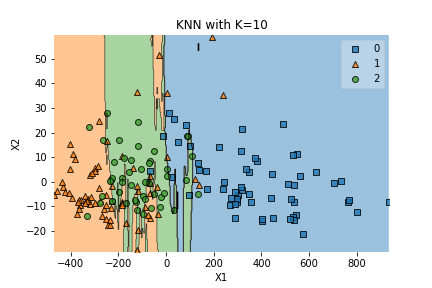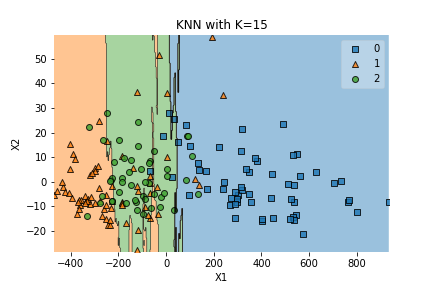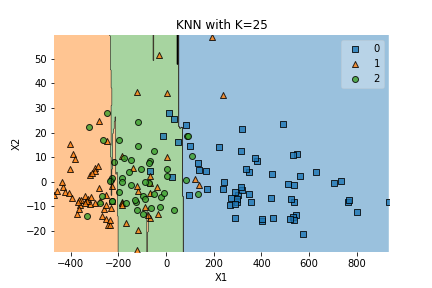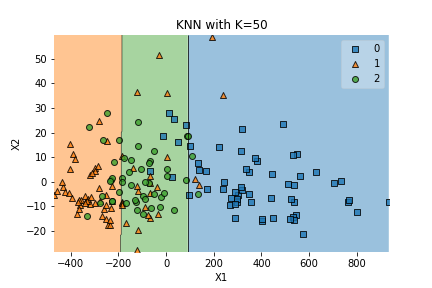# Introduction to Machine Learning: K Nearest Neighbors (KNN)

K Nearest Neighbors or KNN is a standard Machine Learning algorithm used for classification. In KNN, we plot already labeled points with their label and then define decision boundaries based on the value of the hyperparameter “K”. Hyperparameter just means a parameter that we control and can use for tuning. “K” is used to represent how many of the nearest neighbors we should take into account when determining the class of a new point.

For a video version:

For a video animation:

In this post we’ll cover how to do KNN on two datasets, one contrived sample dataset and one more realistic dataset about wine from `sklearn`. For this module we’ll need to install the `sklearn`, `matplotlib`, `mlxtend`, `numpy` and `pandas` libraries. To do so, we can simply run the following line in the command line:

``pip install sklearn matplotlib mlxtend numpy pandas``

As with all of our tutorials, we’re going to start with importing our libraries. We’ll import `matplotlib.pyplot` for plotting, `pandas` for data wrangling, `numpy` for numerical operations, `random` for creating our randomized dataset, `sklearn` for its pre-built KNN, and the `plot_decision_regions` module from `mlxtend.plotting` to plot our KNN regions.

``````import matplotlib.pyplot as plt
import pandas as pd
import random
import numpy as np
from sklearn import datasets, neighbors
from sklearn.decomposition import PCA
from mlxtend.plotting import plot_decision_regions``````

# K Nearest Neighbors on Contrived Random Dataset

Let’s start off our KNN analysis by creating a function that will perform KNN on some dataset with some hyperparameter k. In our function we’ll assume that the first index of the passed in data contains the `x` values and the second index contains the `y` values. Later we’ll simply structure our data this way for ease (also this is how the data is returned from `sklearn` datasets). Then we’ll call the `KNeighborsClassifier` from `sklearn.neighbors` with a parameter of `k` neighbors to make a classifier. We’ll fit our classifier on our `x` and `y` data and then call `plot_decision_regions` to plot the data with the classifier we just fit (that’s what the `clf` parameter is for). Finally we’ll label our plot and show it.

``````def knn(data, k):
x = data
y = data
knn = neighbors.KNeighborsClassifier(n_neighbors=k)
knn.fit(x, y)
plot_decision_regions(x, y, clf=knn)
plt.xlabel('X1')
plt.ylabel('X2')
plt.title(f'KNN with K={str(k)}')
plt.show()``````

## Generate Randomized Data

Now let’s create a dataset and run our function on it. Let’s create two lists, one for the contrived data and one for the contrived targets/classifications. This will create a 2D blob dataset of 100 data points where each feature is uniformly distributed between 0 and 1. We’ll classify `y`, our target, based on the sum of the two randomly generated features. Then we’ll add our contrived data and contrived targets to our `full_example_data` so that the setup matches what the `knn` function we created earlier expects. Finally we’ll simply call the function we created above with some value of `k`, in this example 5, and we should get a plot back.

``````contrived_data = []
contrived_targets = []
samples = 100
# x is two dimensional randomly distributed between 0 and 1
# y is 1 if x1+x2 > 1 and 0 otherwise
for i in range(samples):
x1 = random.uniform(0,1)
x2 = random.uniform(0,1)
y = 1 if x1 + x2 > 1 else 0
contrived_data.append([x1, x2])
contrived_targets.append(y)
full_example_data = [np.array(contrived_data), np.array(contrived_targets)]
# print(full_example_data)
knn(full_example_data, 5)``````

We should get some graph that looks like this:

# K Nearest Neighbors on SKLearn’s Wine Dataset

Now that we have practice setting up an example dataset, let’s also try this out on a more realistic dataset. We already have all the imports set up from above so let’s dive right into the code. We’ll have to make a new KNN function because this dataset doesn’t automatically come with two features for the `x` value. Here we’ll have to use Principal Component Analysis (PCA) to reduce our dimensions into two dimensions just like we did in the K Means example.

Let’s get into our function and how it’s going to differ. You’ll notice most of this function is exactly the same as the function above. Here’s the difference – I’ve added one line that runs PCA on the `x` data passed in. The wine dataset’s `x` data comes with 13 dimensions, and we can only see two so we’re going to need to put it into two dimensions. The only other change is that I also call a `.savefig` function on our plot to save these figures as images. This is because we’re going to use this dataset to explore what different values of `k` can do to our decision boundaries. I want to save the graphs, but feel free to leave it out if you don’t want to save the graphs. (I give this function a slightly different name just to separate it from the other one)

``````def knn_r(data, k):
x = data
y = data
x = PCA(n_components=2).fit_transform(x)
knn = neighbors.KNeighborsClassifier(n_neighbors=k)
knn.fit(x, y)
plot_decision_regions(x, y, clf=knn)
plt.xlabel('X1')
plt.ylabel('X2')
plt.title(f'KNN with K={str(k)}')
plt.savefig(f'KNN with k={str(k)}')
plt.show()``````

After we define our function, there’s a lot less work to do here since we don’t even need to come up with a dataset. We simply call the `load_wine` function from `sklearn` with a `return_X_y` parameter set to True and we’ll get our wine data set back separated into `x` and `y` data points.

``````data = datasets.load_wine(return_X_y=True)
for i in [5, 10, 15, 25, 50]:
knn_r(data, i)``````

Now when we run our function we’ll get to see a bunch of different decision boundaries based on `k`. We should get images that look like the following:K = 5, K= 10, and K = 15 all give us a pretty scattered set of boundaries. They’re pretty rough looking graphs but we can tell that’s because of some outliers in the triangle class. Remember that these are three classes of wine, and no one really knows anything about wine anyway.K = 25 and K = 50 start to give us a more orderly looking decision boundary, but don’t make the mistake of thinking more orderly necessarily equals better. It also looks like there’s a lot more mixup between the classes. We can see that although the triangle class is problematic and has many outliers to begin with, there’s many more incorrectly classified triangles in these two graphs than in the ones with lower K’s. There’s also more incorrectly classified circles, but the square class seems to be doing better.

I hope this gives you a good picture of what K Nearest Neighbors is and what it looks like. The tuning parameter (or hyperparameter) K is critical to getting a good KNN classifier as we’ve seen from the wine dataset example. Which of the K’s is necessarily better? That’s for you to decide based on what you’re doing with the classification and why you’re classifying it. I would say 10 or 15 seem to have the best decision boundaries. We can alternatively improve this by splitting the datasets into train/test and using the train data to create a decision region and the test to test and see how many points are classified correctly or incorrectly, but we’ll have to leave that to a future post.

I run this site to help you and others like you find cool projects and practice software skills. If this is helpful for you and you enjoy your ad free site, please help fund this site by donating below! If you can’t donate right now, please think of us next time.